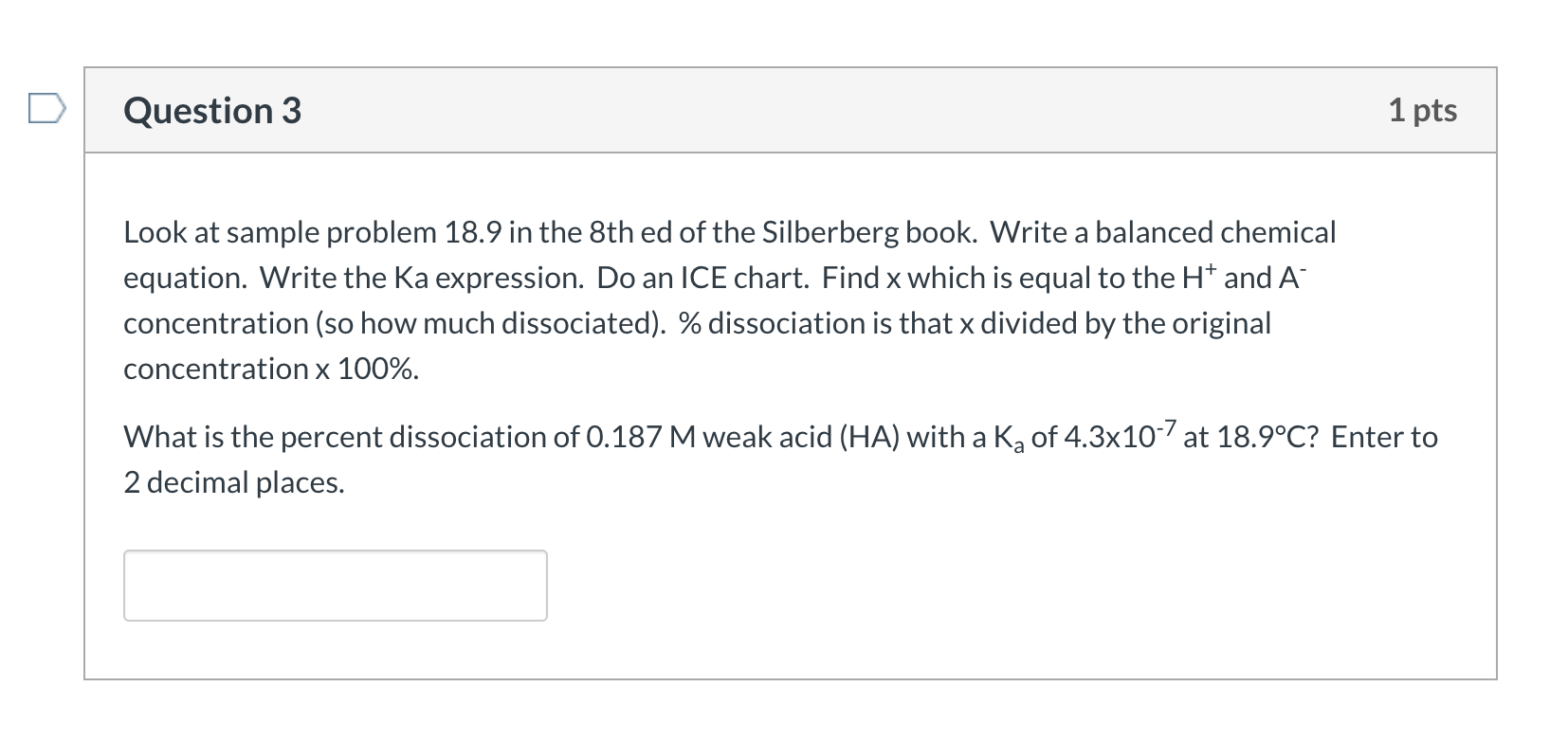Home / Answered Questions / Other / question-3-1-pts-look-at-sample-problem-18-9-in-the-8th-ed-of-the-silberberg-book-write-a-balanced-c-aw120

# (Solved): Question 3 1 Pts Look At Sample Problem 18.9 In The 8th Ed Of The Silberberg Book. Write A Balanced ...Question 3 1 pts Look at sample problem 18.9 in the 8th ed of the Silberberg book. Write a balanced chemical equation. Write the Ka expression. Do an ICE chart. Find x which is equal to the Ht and A concentration (so how much dissociated). % dissociation is that x divided by the original concentration x 100%. What is the percent dissociation of 0.187 M weak acid (HA) with a Ka of 4.3x10-7 at 18.9Â°C? Enter to 2 decimal places.

We have an Answer from Expert